Next: 8.1.1 Entire Layout Up: 8. Simulation Examples Previous: 8. Simulation Examples

# 8.1 Aerial Image Simulation of the Layout of a Multiplexer

The entire layout of the 4:16 multiplexer is shown in Figure 8.1. The aerial images of the following four layers are calculated since these layers are of special interest for lithography (cf. Figure 8.2):
1.
The POLY1 layer defines the transistor channel length and thus carries the smallest features of all layers.
2.
The ACTIVE-AREA layer defines the transistor width and is together with POLY1 of crucial importance for a well operating transistor.
3.
The CONTACT layer defines the contacts between POLY1 and METAL1 as well as between ACTIVE-AREA and METAL1. Among all contact and via layers it has patterns with minimal feature size.
4.
The METAL1 layer is the first metalization layer. Its feature dimensions are thus minimal of all back-end layers.

The other four layers constituting the overall manufacturing process, namely N-WELL, P-IMPLANT, METAL2, and VIA, are omitted since they are less interesting with respect to lithography. The layout is 52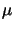x 29m large, whereas the smallest pattern is of size 0.25m (cf. Figure 8.1 and Figure 8.2). The ratio between minimal feature size and lateral x- and y-extensions of the layout is 208 and 116, respectively.

The stepper is a DUV projection printing system with a wavelength of 248 nm, a numerical aperture of NA = 0.6, and a partial coherence factor of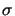= 0.7. Following the Rayleigh criteria given in (2.1) and (2.2), the minimal printable feature size W and the achievable depth of focus  DOF are approximately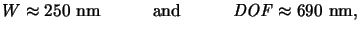(8.1)

whereby for the process parameters k1 = 0.6 and k2 = 1.0 are inserted [11, p. 415]. The stepper is thus capable to print the 0.25m technology, but the required resolution is already critical. Therefore the printing tolerance is small, especially the shallow depth of focus of 690 nm causes problems in a factory floor process. Advanced lithographic techniques such as off-axis apertures and phase-shifting masks enhance the performance considerably. Printing with a reasonably large latitude becomes feasible without changing the core of the stepper, i.e., the wavelength of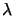= 248 nm and the numerical aperture of NA = 0.6 are kept constant. Before we discuss the simulation results, a few notes on the computational performance of the aerial image module are given.

As can be seen in Figure 8.3 and Figure 8.5 a 57x 33m large simulation area is chosen. The ortho-product-tensor-grid has 1024(210) ticks in x-direction, and 512(29) ticks in y-direction. Approximately 5 grid points per wavelength and thus also per minimal feature size are used. The wavevector sampling of (4.56) yields diffraction orders of | Nx|max = 136 and | Ny|max = 79 that are most obliquely incident on the wafer, i.e.,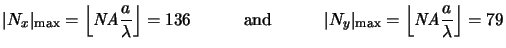(8.2)

are the largest numbers fulfilling (4.58). In total 33736 diffraction orders are imaged by the projection lens since a circular pupil function is assumed, i.e.,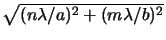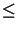NA. The originally proposed source discretization using the same high resolution of/a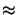4.39 10-3 and/b7.58 10-3 would yield 25321 points (p, q) obeying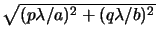NA(cf. Figure 4.8). However, it suffices to take every fourth and second source point in x- and y-direction, respectively, i.e., the source sampling rate is set to sx, p = 4/a and sy, q = 2/b. The aerial image is then built up by the superposition of 2319 coherent contributions (cf. (4.83)). Memory consumption and run-time depend on the simulated layers since each of them carries a different number NPAT of patterns. The actual performance figures are summarized in Table 8.1.

Table 8.1: Performance figures of large area aerial image simulation. The simulations were run on a DEC-600/333 workstation.

 Layer NPAT NTRI Storage CPU ACTIVE-AREA 151 1266 13 MB 36 minutes POLY1 51 440 12 MB 29 minutes CONTACT 1304 2608 14 MB 49 minutes METAL1 165 1558 13 MB 39 minutes

As can be seen, the storage requirements only slightly depend on the number of patterns, whereas the dependence of the run-time is more pronounced. The reason is that the analytical forward transform of the mask transfer function has to be evaluated for every pattern, whereas the costs of the numerical backward transform do not depend on the number of patterns (cf. Section 4.4). This situation can be quantified in a simple form by observing from Table 8.1 that the run-time approximately grows like(8.3)

whereby NTRI is the number of triangles obtained from the decomposition of the layout into triangular-shaped patterns (cf. Section 4.4.1). The first contribution is due to the numerical backward transform and is constant with respect to the number NTRI of triangles. It corresponds for the applied numerical parameters to the minimal run-time occurring for an empty'' layout, i.e., tCPU, min = 25.27 minutes. The second term is due to the analytical forward transform and is thus proportional to NTRI. This shows that the analytical forward transform is advantageous not only because of accuracy but also because of run-time. However, if the number of triangles exceeds a certain number--in our example this critical number is N*TRI2 980--a numerical forward transform would be faster since then the analytical algorithm takes longer than the 25.27 minutes of (8.3). Aliasing errors are then of course introduced (cf. Section 4.4.1). Note that the analytical algorithm can easily be enhanced by searching all equally shaped patterns, transforming them only once, and multiplying this single transformation accordingly to the shift-invariance property of the Fourier transformation, i.e., the basic transformation has to be multiplied with the exponential function exp(j2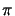(n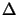x/a + my/b)), wherebyx andy refer to the spatial x- and y -offsets of the patterns, respectively.Next: 8.1.1 Entire Layout Up: 8. Simulation Examples Previous: 8. Simulation Examples
Heinrich Kirchauer, Institute for Microelectronics, TU Vienna
1998-04-17